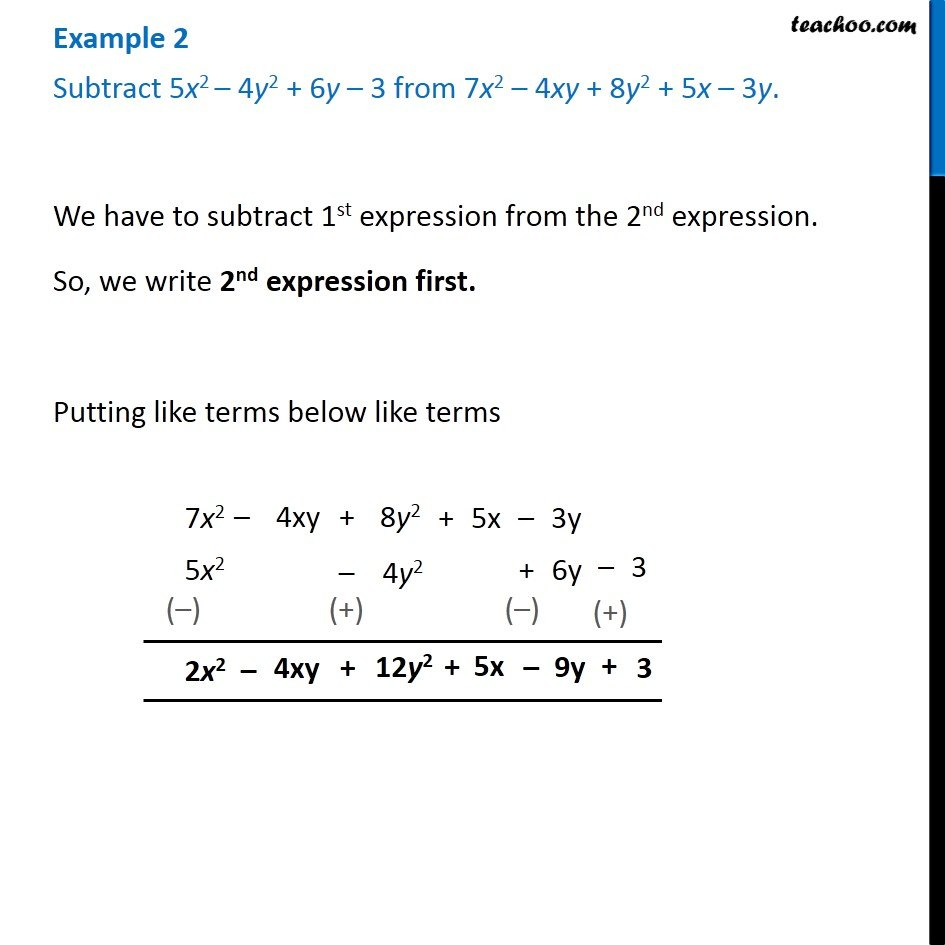1. Chapter 9 Class 8 Algebraic Expressions and Identities
2. Serial order wise
3. Examples

Transcript

Example 2 Subtract 5x2 – 4y2 + 6y – 3 from 7x2 – 4xy + 8y2 + 5x – 3y. We have to subtract 1st expression from the 2nd expression. So, we write 2nd expression first. Putting like terms below like terms

Examples